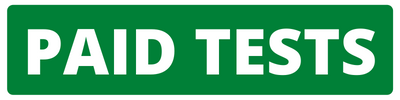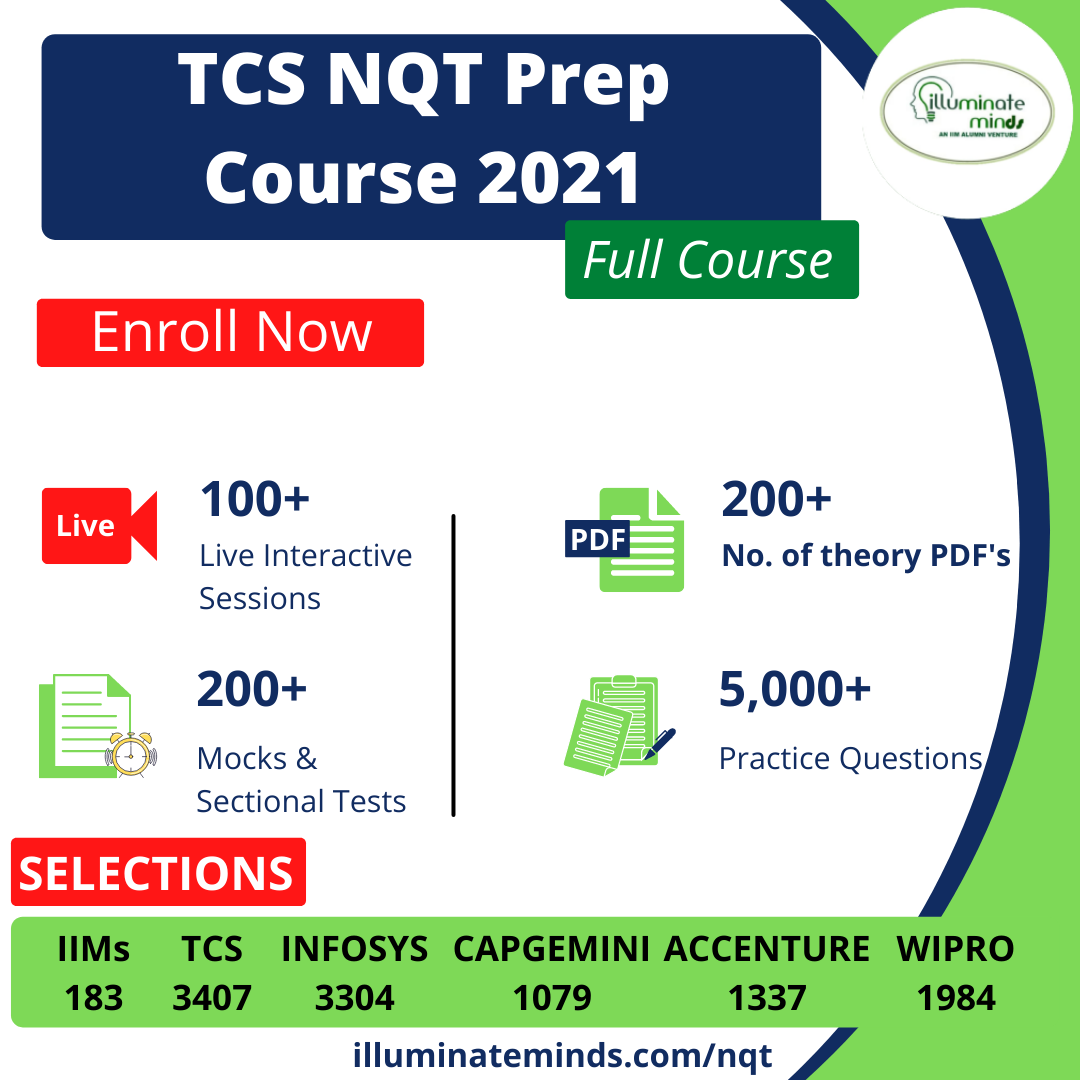CAT | Linear & Quadratic Equations

## CAT | Linear & Quadratic EquationsJobs By Batch
Jobs By Location
Jobs By Degree
Jobs By Branch
IT Jobs
Internships
Govt. Jobs• ##### GE Power Off Campus 2022 | GET  Embedded Systems | BE/B.Tech/ME /M.Tech | 2023/2022/2021/2020 Batch | Chennai• ##### Original Questions of IT Companies| All Topics### Quiz Begins Here

Q #1
:

#### CAT Linear Equations - Counting

3x + 4|y| = 33. How many integer values of (x, y) are possible?

+

Q #2
:

#### CAT Linear Equations - Counting

(|x| - 3) (|y| + 4) = 12. How many pairs of integers (x, y) satisfy this equation?

+

Q #3
:

#### CAT Linear Equations - Integer Values; Modulus

x + |y| = 8, |x| + y = 6. How many pairs of x, y satisfy these two equations?

+

Q #4
:

#### CAT Quadratic Equations - Counting.

What is the number of real solutions of the equation x2 - 7|x| - 18 = 0?

+

Q #5
:

#### CAT Quadratic Equations - Counting

x2 - 9x + |k| = 0 has real roots. How many integer values can 'k' take?

+